Monday 08th August 2022CBSE Guess > Papers > Important Questions > Class XI > 2010 > Maths > Maths By Mr. Anil Kumar Tondak CBSE CLASS XI
Trigonometric Functions Q.1. Find the degree measure corresponding to the following radian measures (Usep = 22/7): (i)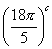(ii) -3)c (iii) 11c Q.2. Find the radian measure corresponding to the following degree measures: (i) -300° (ii) 7° 30° (iii) 125° 30° Q.3. Find the values of the following trigonometric ratios: (i) sin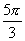(ii) sin 3060° (iii) cosec (-1200°) (iv) tan (-585°) (v) sin 4530° sin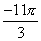Q.4. Find the value of tan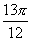. Q.5. Find the value of tan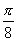. Q.6. Find tan150 and hence show that tan150 + cot150 = 4. Q.7. Evaluate: sin 130° Cos 110° + Cos 130° sin 110° Q.8. Find the value of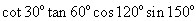. Q.9. Show that: cosec3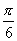,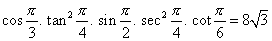. Q.10. Prove that: tan 560 =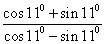Q.11. Prove: tan150 + tan300 + tan150.tan300 = 1. Q.12. Prove that: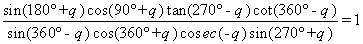Q.13. In a right triangle, the difference between two acute angles is p/18. Express the angles in degrees. Q.14. The minute hand of a watch is 1.5 cm long. How far does its tip move in 40 minutes. Paper By Mr. Anil Kumar Tondak Email Id : [email protected] Ph No.: 9811363962